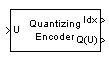# Quantizing Encoder

Quantize signal using partition and codebook

## Library

Source Coding

•## Description

The Quantizing Encoder block quantizes the input signal according to the Partition vector and encodes the input signal according to the Codebook vector. This block processes each vector element independently. The input must be a discrete-time signal. This block processes each vector element independently. For information about the data types each block port supports, see the Supported Data Type table on this page.

The first output is the quantization index. The second output is the quantized signal. The values for the quantized signal are taken from the Codebook vector.

The Quantization partition parameter, P, is a real vector of length n whose entries are in strictly ascending order. The quantization index (second output signal value) corresponding to an input value of x is

• 0 if xP(1)

• m if P(m) < xP(m+1)

• n if P(n) < x

The Quantization codebook parameter, whose length is n+1, prescribes a value for each partition in the quantization. The first element of Quantization codebook is the value for the interval between negative infinity and the first element of P. The second output signal from this block contains the quantization of the input signal based on the quantization indices and prescribed values.

Use the `lloyds` function with a representative sample of your data as training data, to obtain appropriate partition and codebook parameters.

## Parameters

Quantization partition

The vector of endpoints of the partition intervals.

Quantization codebook

The vector of output values assigned to each partition.

Index output data type

Select the output data type.

## Supported Data Type

PortSupported Data Types
U
• Double-precision floating point

• Single-precision floating point

• Signed fixed-point

Idx
• Double-precision floating point

• 8-, 16-, and 32-bit signed integers

• 8-, 16-, and 32-bit unsigned integers

Q(U)
• Double-precision floating point

• Single-precision floating point

• Signed fixed-point

## Pair Block

Quantizing Decoder

## Extended Capabilities

### Functions

Introduced before R2006a